International
Tables for
Crystallography
Volume C
Mathematical, physical and chemical tables
Edited by E. Prince

International Tables for Crystallography (2006). Vol. C, ch. 4.4, p. 453

## Section 4.4.4.5. Measurement of scattering lengths

V. F. Searsg

#### 4.4.4.5. Measurement of scattering lengths

| top | pdf |

The development of modern neutron-optical techniques during the past 25 years has produced a dramatic increase in the accuracy with which scattering lengths can be measured (Koester, 1977; Klein & Werner, 1983; Werner & Klein, 1986; Sears, 1989; Koester, Rauch & Seymann, 1991). The measurements employ a number of effects – mirror reflection, prism refraction, gravity refractometry, Christiansen filter, and interferometry – all of which are based on the fact that the neutron index of refraction, n, is uniquely determined by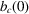through the relation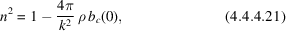in which ρ is the number of atoms per unit volume. Apart from a small (≤0.01%) local-field correction (Sears, 1985, 1989), this expression is exact.

In methods based on diffraction, such as Bragg reflection by powders or dynamical diffraction by perfect crystals, the measured quantity is the unit-cell structure factor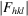. This quantity depends on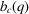in which q is equal to the magnitude of the reciprocal-lattice vector corresponding to the relevant Bragg planes, i.e.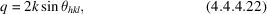where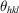is the Bragg angle. In dynamical diffraction measurements, it is usual for the authors to correct their results for electromagnetic interactions so that the published quantity is again. In the past, this correction has not usually been made for the scattering lengths obtained from Bragg reflection by powders. However, these latter measurements are accurate only to ±2 or 3% so that the correction is then relatively unimportant.

The essential point is that all the bound coherent scattering lengths in Table 4.4.4.1with the experimental uncertainties less than 1% representand should therefore be corrected for electromagnetic interactions before being used in the interpretation of neutron diffraction experiments. Failure to make this correction will introduce systematic errors of 0.5 to 2% in the unit-cell structure factors at large q, and corresponding errors of 1 to 4% in the calculated intensities.

Expression (4.4.4.21)assumed that the neutrons and/or the nuclei are unpolarized. If the neutrons and the nuclei are both polarized thenis replaced by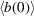, which depends on both the coherent and incoherent scattering lengths. If the coherent scattering length is known, neutron-optical experiments with polarized neutrons and nuclei can then be used to determine the incoherent scattering length (Glättli & Goldman, 1987).

### References

Glättli, H. & Goldman, M. (1987). Nuclear magnetism. Methods of experimental physics, Vol. 23, Neutron scattering Part C, edited by K. Sköld & D. L. Price, pp. 241–286. New York: Academic Press.Google Scholar
Klein, A. G. & Werner, S. A. (1983). Neutron optics. Rep. Prog. Phys. 46, 259–335.Google Scholar
Koester, L. (1977). Neutron scattering lengths and fundamental neutron interactions. Springer Tracts in Modern Physics, Vol. 80, pp. 1–55. Berlin: Springer Verlag.Google Scholar
Koester, L., Rauch, H. & Seymann, E. (1991). Neutron scattering lengths: a survey of experimental data and methods. At. Data Nucl. Data Tables, 49, 65–120.Google Scholar
Sears, V. F. (1985). Local-field refinement of neutron scattering lengths. Z. Phys. A321, 443–449.Google Scholar
Sears, V. F. (1989). Neutron optics. Oxford University Press.Google Scholar
Werner, S. A. & Klein, A. G. (1986). Neutron optics. Methods of experimental physics, Vol. 23, Neutron scattering, Part A, edited by K. Sköld and D. L. Price, pp. 259–337. New York: Academic Press.Google Scholar